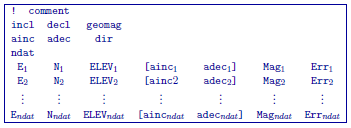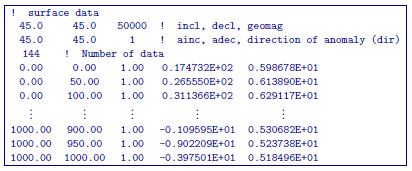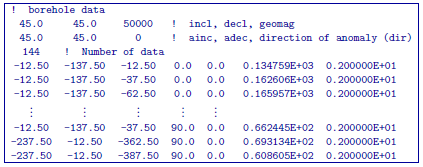# 5.1.3. Magnetic observations: GIF file¶

This file is used to specify the inducing field parameters, anomaly type, observation locations, and the observed magnetic anomalies with estimated standard deviation. The values of parameters specifying the inducing field anomaly type and observation locations are identical to those in file. The output of the forward modelling program has the same structure except that the column of standard deviations for the error is omitted. Lines starting with ! are comments. GIFtools will read either format through when importing a magnetic data set in GIF format. The structure of the GIF-formatted magnetics observations file is:Parameter definitions:

• incl, decl: Inclination and declination of the inducing magnetic field. The declination is specified positive east with respect to the northing used in the mesh and locations files. The inclination is positive down.

• geomag: Strength of the inducing field in nanoTesla (nT).

• ainc, adec, idir: Inclination and declination of the anomaly projection. Set idir=0 for a multi-component dataset and idir=1 for a single component dataset where all the observations have the same inclination and declination of the anomaly projection.

• ndat: Number of observations. When single component data are specified the number of observations is equal to the number of data locations. When multi-component data are specified the number of observations will exceed the number of data locations. For example, if three-component data are specified at $$N$$ locations, the number of observations is $$3N$$.

• E, N, ELEV: Easting, northing, and elevation of the observation measured in meters. Elevation should be above the topography for surface data, and below the topography for borehole data. The observation locations can be listed in any order.

• (ainc$$_{n}$$, adec$$_{n}$$): Inclination and declination of the anomaly projection for nth observation. This is used only when idir=0. The brackets $$[\cdots]$$ indicate that these two field are optional depending upon the value of idir.

• Mag$$_n$$: Magnetic anomaly data, measured in nT.

• Err$$_n$$: Standard deviation of Mag$$_n$$. This represents the absolute error. It must be positive and non-zero.

NOTE: It should be noted that the data are extracted anomalies which are derived by removing the regional from the field measurements. It is crucial that the data be prepared as such. The total field anomaly is calculated when ainc=incl and adec=decl. An example is inputting the vertical field anomaly, Bz, calculated by setting ainc=90 and adec=0. The easting and northing components are respectively given by the inclination and declination pairs (0, 90) and (0, 90). The user can specify other (ainc, adec) pairs to calculate the other anomaly components such as the Bx or By. Easting, northing, and elevation information should be in the same coordinate system as defined in the mesh.

## 5.1.3.1. Examples¶

The following are two examples of data files. The first example file specifies a set of total field anomaly data, and the second example file provides a set of multi-component borehole data.

Example 1: single-component dataExample 2: Multi-component data